Case Based Questions Test: Real Numbers - 2

# Case Based Questions Test: Real Numbers - 2 - Class 10

Test Description

## 10 Questions MCQ Test Mathematics (Maths) Class 10 - Case Based Questions Test: Real Numbers - 2

Case Based Questions Test: Real Numbers - 2 for Class 10 2023 is part of Mathematics (Maths) Class 10 preparation. The Case Based Questions Test: Real Numbers - 2 questions and answers have been prepared according to the Class 10 exam syllabus.The Case Based Questions Test: Real Numbers - 2 MCQs are made for Class 10 2023 Exam. Find important definitions, questions, notes, meanings, examples, exercises, MCQs and online tests for Case Based Questions Test: Real Numbers - 2 below.
Solutions of Case Based Questions Test: Real Numbers - 2 questions in English are available as part of our Mathematics (Maths) Class 10 for Class 10 & Case Based Questions Test: Real Numbers - 2 solutions in Hindi for Mathematics (Maths) Class 10 course. Download more important topics, notes, lectures and mock test series for Class 10 Exam by signing up for free. Attempt Case Based Questions Test: Real Numbers - 2 | 10 questions in 20 minutes | Mock test for Class 10 preparation | Free important questions MCQ to study Mathematics (Maths) Class 10 for Class 10 Exam | Download free PDF with solutions
 1 Crore+ students have signed up on EduRev. Have you?
Case Based Questions Test: Real Numbers - 2 - Question 1

### Direction: Read the following text and answer the following questions on the basis of the same:A seminar is being conducted by an Educational Organisation, where the participants will be educators of different subjects. The number of participants in Hindi, English and Mathematics are 60, 84 and 108 respectively.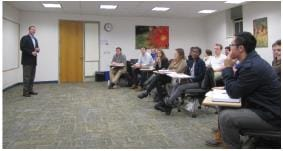108 can be expressed as a product of its primes as

Detailed Solution for Case Based Questions Test: Real Numbers - 2 - Question 1
All even numbers except 2 are composite numbers, so 108 is a composite number.

Therefore, we can conclude that 108 can be expressed as a product of prime factors by 22 × 33.

Case Based Questions Test: Real Numbers - 2 - Question 2

### Direction: Read the following text and answer the following questions on the basis of the same:A seminar is being conducted by an Educational Organisation, where the participants will be educators of different subjects. The number of participants in Hindi, English and Mathematics are 60, 84 and 108 respectively.What is the minimum number of rooms required during the event?

Detailed Solution for Case Based Questions Test: Real Numbers - 2 - Question 2
Minimum no. of rooms required are total number of students divided by number of students in each room.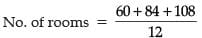= 21

Case Based Questions Test: Real Numbers - 2 - Question 3

### Direction: Read the following text and answer the following questions on the basis of the same:A seminar is being conducted by an Educational Organisation, where the participants will be educators of different subjects. The number of participants in Hindi, English and Mathematics are 60, 84 and 108 respectively.The product of HCF and LCM of 60,84 and 108 is

Detailed Solution for Case Based Questions Test: Real Numbers - 2 - Question 3
60 = (22 × 3 × 5)

84 = (22 × 3 × 7)

108 = (22 × 33)

HCF(60, 84,108) = (22 × 3) = 12

LCM =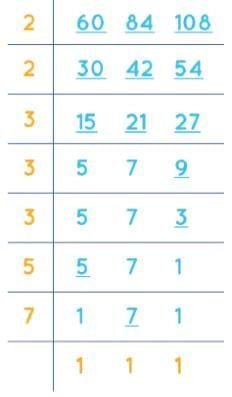LCM of 60, 84, and 108 is 3780.

Product of HCF x LCM = 3780 x 12 = 45360

Case Based Questions Test: Real Numbers - 2 - Question 4

Direction: Read the following text and answer the following questions on the basis of the same:

A seminar is being conducted by an Educational Organisation, where the participants will be educators of different subjects. The number of participants in Hindi, English and Mathematics are 60, 84 and 108 respectively.In each room the same number of participants are to be seated and all of them being in the same subject, hence maximum number participants that can accommodated in each room are

Detailed Solution for Case Based Questions Test: Real Numbers - 2 - Question 4
No. of participants seated in each room would be HCF of all the three values above.

60 = 2 × 2 × 3 × 5

84 = 2 × 2 × 3 × 7

108 = 2 × 2 × 3 × 3 × 3

Hence, HCF = 12.

Case Based Questions Test: Real Numbers - 2 - Question 5

Direction: Read the following text and answer the following questions on the basis of the same:

A seminar is being conducted by an Educational Organisation, where the participants will be educators of different subjects. The number of participants in Hindi, English and Mathematics are 60, 84 and 108 respectively.The LCM of 60, 84 and 108 is

Detailed Solution for Case Based Questions Test: Real Numbers - 2 - Question 5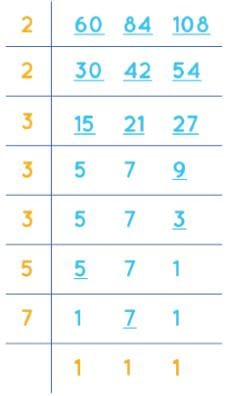LCM = 2 x 2 x 3 x 3 x 3 x 5 x 7

LCM = 3780

Therefore, LCM of 60, 84, and 108 is 3780.

Case Based Questions Test: Real Numbers - 2 - Question 6

Direction: Read the following text and answer the following questions on the basis of the same:

A Mathematics Exhibition is being conducted in your School and one of your friends is making a model of a factor tree. He has some difficulty and asks for your help in completing a quiz for the audience.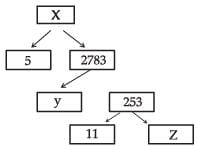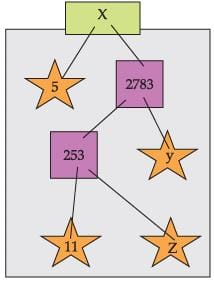The prime factorisation of 13915 is

Detailed Solution for Case Based Questions Test: Real Numbers - 2 - Question 6

Using the prime factorization tree, we have

∴ 13915 = 5 × 11 × 11 × 23 = 5 × 112 × 23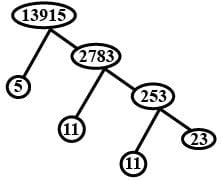Case Based Questions Test: Real Numbers - 2 - Question 7

Direction: Read the following text and answer the following questions on the basis of the same:

A Mathematics Exhibition is being conducted in your School and one of your friends is making a model of a factor tree. He has some difficulty and asks for your help in completing a quiz for the audience.What will be the value of y?

Detailed Solution for Case Based Questions Test: Real Numbers - 2 - Question 7
2783 = y × 253

y = 2783/253

y = 11

Case Based Questions Test: Real Numbers - 2 - Question 8

Direction: Read the following text and answer the following questions on the basis of the same:

A Mathematics Exhibition is being conducted in your School and one of your friends is making a model of a factor tree. He has some difficulty and asks for your help in completing a quiz for the audience.According to Fundamental Theorem of Arithmetic 13915 is a

Detailed Solution for Case Based Questions Test: Real Numbers - 2 - Question 8
The prime factorization of 13,915 is 5 × 112 × 23.

Since it has a total of 4 prime factors, 13,915 is a composite number.

Case Based Questions Test: Real Numbers - 2 - Question 9

Direction: Read the following text and answer the following questions on the basis of the same:

A Mathematics Exhibition is being conducted in your School and one of your friends is making a model of a factor tree. He has some difficulty and asks for your help in completing a quiz for the audience.What will be the value of x?

Detailed Solution for Case Based Questions Test: Real Numbers - 2 - Question 9
x = 2783 × 5

x = 13915

Case Based Questions Test: Real Numbers - 2 - Question 10

Direction: Read the following text and answer the following questions on the basis of the same:

A Mathematics Exhibition is being conducted in your School and one of your friends is making a model of a factor tree. He has some difficulty and asks for your help in completing a quiz for the audience.What will be the value of z?

Detailed Solution for Case Based Questions Test: Real Numbers - 2 - Question 10
253 = 11 × z

z = 253/11

z = 23

## Mathematics (Maths) Class 10

115 videos|478 docs|129 tests
Information about Case Based Questions Test: Real Numbers - 2 Page
In this test you can find the Exam questions for Case Based Questions Test: Real Numbers - 2 solved & explained in the simplest way possible. Besides giving Questions and answers for Case Based Questions Test: Real Numbers - 2, EduRev gives you an ample number of Online tests for practice

## Mathematics (Maths) Class 10

115 videos|478 docs|129 tests